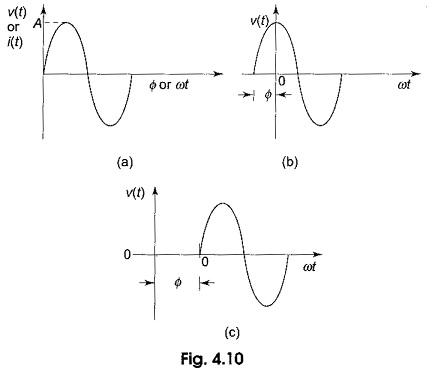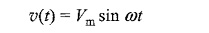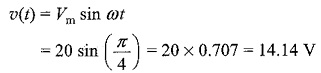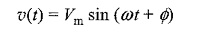## Sine Wave Equation:

A Sine Wave Equation is graphically represented as shown in Fig. 4.10(a). The amplitude of a sine wave is represented on vertical axis. The angular measurement (in degrees or radians) is represented on horizontal axis. Amplitude A is the maximum value of the voltage or current on the Y-axis.In general, the sine wave is represented by the equationThe above Sine Wave Equation states that any point on the sine wave represented by an instantaneous value υ(t) is equal to the maximum value times the sine of the angular frequency at that point.

For example, if a certain sine wave voltage has peak value of 20 V, the instantaneous voltage at a point π/4 radians along the horizontal axis can be calculated asWhen a sine wave is shifted to the left of the reference wave by a certain angle Φ, as shown in Fig. 4.10 (b), the general expression can be written asWhen a sine wave is shifted to the right of the reference wave by a certain angle Φ, as shown in Fig. 4.10(c), the general expression is

Scroll to Top